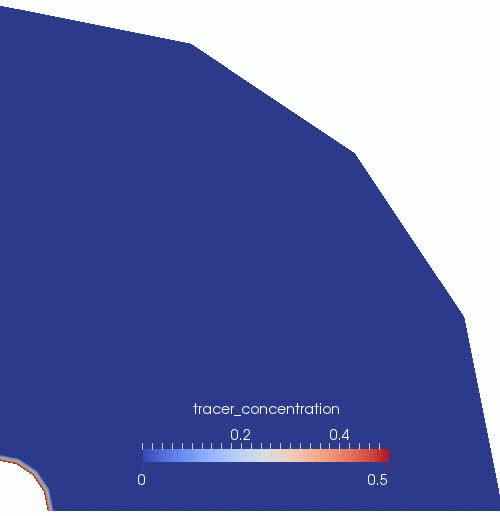# Porous Flow Tutorial Page 06. Adding a tracer

On this Page we will depart from PorousFlowBasicTHM and use PorousFlowFullySaturated. This action employs mass lumping but no numerical stabilization by default. Therefore, the results will be slightly different than those achieved by PorousFlowBasicTHM (no lumping or stabilization) and from the remainder of PorousFlow (mass lumping and either full upwinding or TVD stabilization).

The reason for using PorousFlowFullySaturated is that it allows multi-component physics to be studied. Denoting the mass fraction by (where ), the fluid equations are (1) In this equation the fluid density, , is bracketed, because the user has the option of including it or not using the multiply_by_density flag. The advantage of setting multiply_by_density = false is that the equations become more linear, but it means that care must be taken when using other parts of PorousFlow. For instance, the PorousFlowMass Postprocessor is measuring fluid mass, not fluid volume.

Tracers may now be incorporated into the simulation. A tracer_concentration variable is defined, and set to initial conditions where it is 0.5 at the injection area, and zero elsewhere. Modifying the isothermal simulation of Page 01, PorousFlowFullySaturated is provided with its name:

[Variables]
[./porepressure]
[../]
[./tracer_concentration]
[../]
[]

[ICs]
[./tracer_concentration]
type = FunctionIC
function = '0.5*if(x*x+y*y<1.01,1,0)'
variable = tracer_concentration
[../]
[]

[PorousFlowFullySaturated]
porepressure = porepressure
coupling_type = Hydro
gravity = '0 0 0'
fp = the_simple_fluid
mass_fraction_vars = tracer_concentration
[]

(modules/porous_flow/examples/tutorial/06.i)

To encourage flow, rather than a rapid convergence to a more-or-less steady-state situation, the outer boundary is fixed to . The tracer concentration is fixed to 0.5 at the injection area.

[BCs]
[./constant_injection_porepressure]
type = PresetBC
variable = porepressure
value = 1E6
boundary = injection_area
[../]
[./constant_outer_porepressure]
type = PresetBC
variable = porepressure
value = 0
boundary = rmax
[../]
[./injected_tracer]
type = PresetBC
variable = tracer_concentration
value = 0.5
boundary = injection_area
[../]
[]

(modules/porous_flow/examples/tutorial/06.i)

The only other changes are that the PorousFlowConstantBiotModulus Material is now not needed, and that now a nodal porosity is needed in addition to the one evaluated at the quadpoints, since Eq. (1) is lumped to the nodes:

[Materials]
[./porosity]
type = PorousFlowPorosity
porosity_zero = 0.1
[../]

(modules/porous_flow/examples/tutorial/06.i)

If multiply_by_density = true then the arguments in Page 02 concerning the magnitude of the residual, and hence the size of the nonlinear absolute-tolerance must be modified. Specifically, the fluid residuals now get multiplied by the density (which is the default and most common use-case in PorousFlow). So in this problem, the fluid residual is approximately (2) (since the fluid density is approximately 1000kg.m). The nl_abs_tol is therefore

[Executioner]
type = Transient
solve_type = Newton
end_time = 1E6
dt = 1E5
nl_rel_tol = 1E-14
[]

(modules/porous_flow/examples/tutorial/06.i)

An animation of the results is shown in Figure 1.Figure 1: Darcy flow vectors and tracer evolution in the borehole-aquifer-caprock system.

As you gain experience with PorousFlow, you will realise that this simulation contains several defects.

## Boundary conditions

The most important (potential) problem lies with the boundary conditions. Physically they are saying "add or remove fluid to keep the porepressure or tracer concentration fixed". In more complex simulations this can be completely disasterous. There may not be the correct fluid component and fluid phase present to remove, and this causes extremely poor nonlinear convergence. It is really important to understand this fully.

PorousFlowFullySaturated associates the variable with the mass-balance equation for , and the variable with the mass-balance equation for the final mass fraction (). (This is not obvious unless you read the code. In simulations that don't use Actions it will be obvious because you will have to write the input-file Kernels block yourself.) Hence the BCs that have variable = porepressure:

  [./constant_injection_porepressure]
type = PresetBC
variable = porepressure
value = 1E6
boundary = injection_area
[../]
[./constant_outer_porepressure]
type = PresetBC
variable = porepressure
value = 0
boundary = rmax
[../]

(modules/porous_flow/examples/tutorial/06.i)

are saying "add or remove the final mass fraction () to keep the porepressure fixed"; while the BCs that have variable = tracer_concentration:

  [./injected_tracer]
type = PresetBC
variable = tracer_concentration
value = 0.5
boundary = injection_area
[../]

(modules/porous_flow/examples/tutorial/06.i)

are saying "add or remove the tracer so that its concentration remains fixed". In complex multi-phase, multi-component models this can be a real "gotcha" and a PorousFlowSink is recommended.

## Lack of numerical stabilization

Broadly speaking, the animation shows the expected behaviour, but what are those "stripes" of low and high concentration towards the end of the simulation? This is caused by the lack of numerical stabilization. As mentioned in the introduction to this Page, stabilization is standard in most PorousFlow simulations, but is not used in the above simulation. In fact, if you run the simulation yourself, you'll see that tracer_concentration does not even lie within its expected range of !

The "stripes" are removed by numerical stabilization, but this comes at a cost. PorousFlow offers two types of numerical stabilization. Full-upwinding is excellent at eliminating overshoots and undershoots, and the convergence speed is also excellent, but it introduces extra diffusion, so that sharp fronts are not maintained! On the other hand, KT stabilization also eliminates overshoots and undershoots and introduces very little or zero extra diffusion so that sharp fronts are maintained as well as without any numerical stabilization, but its convergence speed and runtime are always greater. Further details regarding the diffusion of fronts may be found in the numerical diffusion page.

It is not possible to use full-upwinding with the PorousFlowFullySaturated Action, but KT stabilization may be easily introduced:

[PorousFlowFullySaturated]
porepressure = porepressure
coupling_type = Hydro
gravity = '0 0 0'
fp = the_simple_fluid
mass_fraction_vars = tracer_concentration
stabilization = KT
flux_limiter_type = superbee

(modules/porous_flow/examples/tutorial/06_KT.i)

Users are strongly encouraged to experiment with KT stabilization and report their findings back to the moose-users google group, since this stabilization is still at the development stage.